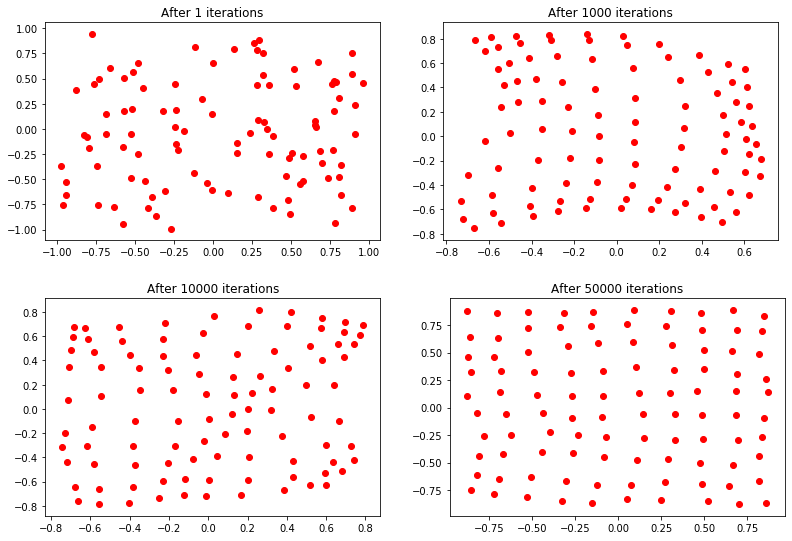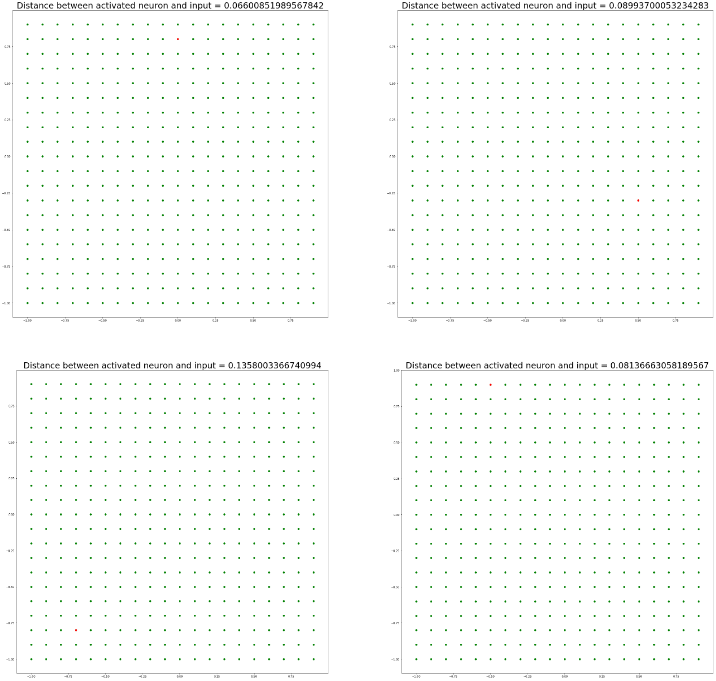# ANN – Implementation of Self Organizing Neural Network (SONN) from Scratch

Prerequisite: ANN | Self Organizing Neural Network (SONN) Learning Algorithm

To implement a SONN, here are some essential consideration-

1. Construct a Self Organizing Neural Network (SONN) or Kohonen Network with 100 neurons arranged in a 2-dimensional matrix with 10 rows and 10 columns
2. Train the network with 1500 2-dimensional input vectors randomly generated in the interval between -1 and +1
3. Select initial synaptic weights randomly in the same interval -1 and +1
4. Assign learning rate parameteris equal to 0.1
5. Objective is to classify 2-dimensional input vectors such that each neuron in the network should respond only to the input vectors occurring in its region
6. Test the performance of the self organizing neurons using the following Input vectors:Python Implementation of SONN:

 # Importing Libraries   import math  from tqdm.notebook import tqdm  import matplotlib.pyplot as plt  import numpy as np     # Generating Data : using uniform random number generator   data_ = np.random.uniform(-1, 1, (1500, 2))  # print(data_.shape)     # Hyperparameter Initialization  x, y = 10, 10        # dimensions of Map  sigma = 1.           # spread of neighborhood  learning_rate = 0.5  # learning rate  epochs = 50000       # no of iterations  decay_parameter = epochs / 2   # decay parameter     # Activation map and Assigning Weights  # using random number generation   activation_map = np.zeros((x, y))  weights = 2 * (np.random.ranf((x, y, data_.shape)) - 0.5)  # print(weights.shape)     # Define Neighborhood Region  neighbour_x = np.arange(x)  neighbour_y = np.arange(y)     # Function: decay_learning_rate_sigma  def decay_learning_rate_sigma(iteration):    learning_rate_ = learning_rate/(1 + iteration / decay_parameter)    sigma_ = sigma / (1 + iteration / decay_parameter)       return learning_rate_, sigma_     # Function: to get winner neuron  def get_winner_neuron(x):    s = np.subtract(x, weights) # x - w    it = np.nditer(activation_map, flags =['multi_index'])    while not it.finished:        # || x - w ||        activation_map[it.multi_index] = np.linalg.norm(s[it.multi_index])          it.iternext()       return np.unravel_index(activation_map.argmin(), activation_map.shape)     # Update weights  def update_weights(win_neuron, inputx, iteration):    # decay learning rate and sigma    learning_rate_, sigma_ = decay_learning_rate_sigma(iteration)       # get neighborhood about winning neuron (Mexican hat function)    d = 2 * np.pi * (sigma_**2)    ax = np.exp(-1 * np.square(neighbour_x - win_neuron) / d)    ay = np.exp(-1 * np.square(neighbour_y - win_neuron) / d)       neighborhood = np.outer(ax, ay)       it = np.nditer(neighborhood, flags = ['multi_index'])    while not it.finished:        weights[it.multi_index] += learning_rate_ * neighborhood[it.multi_index]                                   * (inputx - weights[it.multi_index])        it.iternext()     # Training model: Learning Phase  for epoch in tqdm(range(1, epochs + 1)):    np.random.shuffle(data_)    idx = np.random.randint(0, data_.shape)    win_neuron = get_winner_neuron(data_[idx])    update_weights(win_neuron, data_[idx], epoch)       if epoch == 1 or epoch == 100 == 0 or epoch == 1000 or       epoch == 10000 or epoch == 50000:      plot_x = []      plot_y = []         for i in range(weights.shape):        for j in range(weights.shape):          plot_x.append(weights[i][j])          plot_y.append(weights[i][j])         plt.title('After ' + str(epoch) + ' iterations')      plt.scatter(plot_x, plot_y, c = 'r')      plt.show()      plt.close()

Output:# Testing the model performance  test_inputs = np.array([[0.1, 0.8], [0.5, -0.2], [-0.8, -0.9], [-0.6, 0.9]])   # print(test_inputs.shape)      # The plots below depict the working of this Kohonen Network on   # given test inputs [0.1, 0.8], [0.5, -0.2], [-0.8, -0.9], [-0.6, 0.9]  for i in range(test_inputs.shape):    test_input = test_inputs[i, :]    win_neuron = get_winner_neuron(test_input)       plot_x = np.arange(-1, 1, 0.1)    plot_y = np.arange(-1, 1, 0.1)    xx, yy = np.meshgrid(plot_x, plot_y)       coordx, coordy = weights[win_neuron][win_neuron],                              weights[win_neuron][win_neuron]    dist = math.sqrt((coordx-test_input)**2 + (coordy - test_input)**2)    coordx = round(coordx, 1)    coordy = round(coordy, 1)       plt.figure(figsize =(20, 20))    plt.title("Distance between activated neuron and input = " +              str(dist), fontsize = 30)    plt.scatter(xx, yy, c = 'g')    plt.scatter(coordx, coordy, c = 'r')    plt.show()    plt.close()

Output:All the 10×10 neurons are represented by green color. The nearest neuron which is activated by the test input is shown with red color. Here, the euclidean distances between activated neurons and test inputs are also shown in these plots. Respective neuron will respond for each of the test input vector samples.

My Personal Notes arrow_drop_upCheck out this Author's contributed articles.

If you like GeeksforGeeks and would like to contribute, you can also write an article using contribute.geeksforgeeks.org or mail your article to contribute@geeksforgeeks.org. See your article appearing on the GeeksforGeeks main page and help other Geeks.

Please Improve this article if you find anything incorrect by clicking on the "Improve Article" button below.